## Data Communications Networking Chapter 3, Problem 48E

Question: What is the total delay (latency) for a frame of size 5 million bits that is being sent on a link with 10 routers each having a queuing time of 2 μs and a processing time of 1 μs. The length of the link is 2000 Km. The speed of light inside the link is 2 × 108 m/s. The link has a bandwidth of 5 Mbps. Which component of the total delay is dominant? Which one is negligible?

Solution:

Given data:

Distance (length of the link) =2000 Km

=2000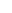103m

Propagation speed=2108m/s

Frame size=5million bits

=5000000bits

The Bandwidth=5Mbps

=5106 bps (1Mbps=106 bps)

Queuing time=102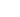(10 routers each having a queuing time of 2)

=20Processing delay=101(10 routers each having a processing time of 1)

=10Total delay (Latency) = Propagation time + Transmission time + Queuing time

+ Processing delay.

Propagation time=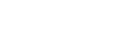=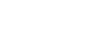= 0.01s

Therefore, Propagation time=0.01s

Transmission time=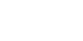=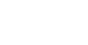= 1s

Therefore, Transmission time=1s

Queuing time=102=10210-6s (1=10-6s)

=2010-6s

= 0.00002s

Therefore, Queuing time=0.00002s

Processing delay=101=1010-6s (1=10-6s)

Therefore, Processing delay=0.000010s

Therefore, Total delay (Latency) =0.01+1+0.000020+0.000010

=1.01003s